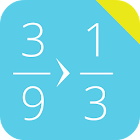Simplify Fractions Calculator

• 검색 유형

모든 Android 애플리케이션 카테고리

모든 Android 게임 카테고리# Simplify Fractions Calculator

by: 1.4K 7.6

7.6 사용자
평가 등급

## 스크린샷

설명

This free math calculator is able to simplify fractions. Just enter the fraction, click the button and the app will simplify.

Best mathematical tool for school and college! If you are a student, it will helps you to learn arithmetics and calculations with fraction numbers.

Note: A common fraction can be reduced to lowest terms by dividing both the numerator and denominator by their greatest common divisor.

: 1.394 리뷰

"우수"

7.6